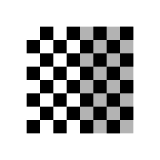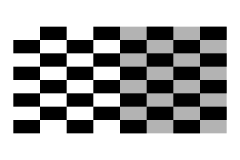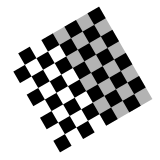Learning Objectives

• Learn how to use Affine transforms

• Differentiate translate, rotate, skew, shear

# Affine Transforms#

Affine transformations allows us to use simple systems of linear equations to manipulate any point or set of points. It allows us to move, stretch, or even rotate a point or set of points. In the case of GIS, it is used to distort raster data, for instance satellite imagery, to fit a new projection or CRS.

First some general properties of affine transforms:

• Preserves

• Points, straight linear & planes

• Sets of parallel lines

• Ratio of distances between points on same straight line

• Distorts

• Angle between lines

• Distance between points

## Types of Transformations#

There are four core ways that you can manipulate an image. These are called “Transforms”:

Transform

Description

Example

Translation

Moves a set of points some fixed distance in the x and y planeScale

Increases or decreases the scale, or distance between points in the x and y planeRotate

Rotates points around the origin, or some defined axisShear

Shifts points in proportion to any given points x and y coordinateImage Credit: Wikipedia

In combination we can warp any point, or set of points (e.g. raster image) into a new projection. This is the equivalent of reprojection for vector data. In order to implement these transforms we will need to learn about the math behind Affine Transforms! Yeah!

### Simple Transform Examples#

To simplify things first lets think about how to do transformations of a single point in a 2d space.

For any location $$\mathbf{x} = (x,y)$$ we can transform $$\mathbf{x}$$ to $$\mathbf{\acute{x}}$$ using simple linear adjustments. Here we can think of point $$\mathbf{x}$$ as being stored as an array:

$\begin{split} \begin{eqnarray} \mathbf{x} = \left[ \begin{array}{cc|r}x \\ y \end{array} \right] \mbox{can be transformed to } \mathbf{\acute{x}} = \left[ \begin{array}{cc|r}\acute{x} \\ \acute{y} \end{array} \right] \end{eqnarray} \end{split}$

From here we can transform the values in the $$x$$ and $$y$$ position using scaler values $$a$$ through $$f$$:

$\begin{split} \begin{eqnarray} \mathbf{\acute{x}} = \left[ \begin{array}{cc|r}ax+by+c \\ dx+ey+f \end{array} \right] \end{eqnarray} \end{split}$

Note

Looking at the formula above, we are adjusting the value of $$x$$ with $$ax+by+c$$, so $$x$$ can be multiplied (scaled) by some value $$a$$, it can also be scaled based on the $$y$$ value with $$+ by$$, or simply adjusted up or down by the value $$+ c$$

To understand how this works, let’s walk through the basic transformations of $$\mathbf{x}$$:

If we multiply $$x$$ and $$y$$ by one, by setting $$a,e = 1$$, and zero out the effect of the other axis, by setting $$b,d = 0$$, we have a simple case of translation, where $$x$$ moves right by $$c$$ and $$y$$ up by the value of $$f$$:

$\begin{split} \begin{eqnarray} \mathbf{\acute{x}} = \left[ \begin{array}{cc|r}1x+0y+c \\ 0x+1y+f \end{array} \right] \end{eqnarray} \end{split}$

We can scale $$x$$ and $$y$$ by setting $$a$$ and $$e$$ to 0.5 (or some fraction), and setting all other values to zero ($$b,d,c,f = 0$$):

$\begin{split} \begin{eqnarray} \mathbf{\acute{x}} = \left[ \begin{array}{cc|r}ax \\ ey \end{array} \right] \end{eqnarray} \end{split}$

We can rotate a point around the origin by setting $$a,e = \cos\theta$$, $$b = -\sin\theta$$ and $$c,f = 0$$:

$\begin{split} \begin{eqnarray} \mathbf{\acute{x}} = \left[ \begin{array}{cc|r} x\cos\theta - y\sin\theta \\ x\sin\theta+y\cos\theta \end{array} \right] \end{eqnarray} \end{split}$

where $$\theta$$ is the angle of rotation (counterclockwise) around the origin.

Finally by adjusting $$x$$ based on the value of $$y$$ (and visa versa), we can achieve a shear transform:

$\begin{split} \begin{eqnarray} \mathbf{\acute{x}} = \left[ \begin{array}{cc|r} x+by \\ y+dx \end{array} \right] \end{eqnarray} \end{split}$

### Transforming Matrices#

It is often convenient to represent these equations as matrices. This allows us to easily chain together a series of operations. We can represent our transformed point $$\mathbf{\acute{x}}$$ as follows:

$\begin{split} \begin{eqnarray} \left[ \begin{array}{cc|r} \acute{x} \\ \acute{y} \end{array} \right] = \left[ \begin{array}{cc|r} ax+by \\ dx+ey \end{array} \right] = \left[ \begin{array}{cc|r} a \ \ b \\ d \ \ e \end{array} \right] \left[ \begin{array}{cc|r} x \\ y \end{array} \right] \end{eqnarray} \end{split}$

Note

If you aren’t familiar with how matrix multiplication works please watch this.

In this new context we can easily do a scale, rotate or shear transform by replacing the matrix of $$a,b,d,e$$ with:

$\begin{split} \begin{eqnarray} \mbox{Rotate: } \left[ \begin{array}{cc|r} \cos\theta \ \ -\sin\theta \\ \sin\theta \ \ \cos\theta \end{array} \right] \end{eqnarray} \end{split}$
$\begin{split} \begin{eqnarray} \mbox{Scale: } \left[ \begin{array}{cc|r} S_{x} \ \ 0 \\ 0 \ \ S_{y} \end{array} \right] \end{eqnarray} \end{split}$
$\begin{split} \begin{eqnarray} \mbox{Shear: } \left[ \begin{array}{cc|r} 1 \ \ r_{x} \\ r_{y} \ \ 1 \end{array} \right] \end{eqnarray} \end{split}$

But wait, what about the easiest transform, “translation”? Unfortunately that makes things a little more complicated! But not that complicated.

In order to be able to perform a translate in matrix form we need to extend our matrices, adding one row along the bottom. In the following form we can now perform all the basic transformations to calculate $$\mathbf{\acute{x}}$$:

$\begin{split} \begin{eqnarray} \left[ \begin{array}{cc|r} \acute{x} \\ \acute{y} \\ 1 \end{array} \right] = \left[ \begin{array}{cc|r} a \ \ b \ \ c \\ d \ \ e \ \ f \\ 0 \ \ 0 \ \ 1 \end{array} \right] \left[ \begin{array}{cc|r} x \\ y \\ 1 \end{array} \right] = \left[ \begin{array}{cc|r} ax+by+c \\ dx+ey+f \\ 1 \end{array} \right] \end{eqnarray} \end{split}$

Now that we have scalers $$c$$ and $$f$$ all the transforms are possible. We do however, need to update our previous operations:

$\begin{split} \begin{eqnarray} \mbox{Translate: } \begin{bmatrix} 1 & 0 & \Delta x \\ 0 & 1 & \Delta y \\ 0 & 0 & 1 \end{bmatrix} \end{eqnarray} \end{split}$

Where $$\Delta x$$ shifts in the $$x$$ axis and $$\Delta y$$ determines the shift in the $$y$$ axis.

$\begin{split} \begin{eqnarray} \mbox{Rotate: } \begin{bmatrix} \cos\theta & -\sin\theta & 0 \\ \sin\theta & \cos\theta & 0 \\ 0 & 0 & 1 \end{bmatrix} \end{eqnarray} \end{split}$
$\begin{split} \begin{eqnarray} \mbox{Scale: } \begin{bmatrix} S_{x} & 0 & 0 \\ 0 & S_{y} & 0 \\ 0 & 0 & 1 \end{bmatrix} \end{eqnarray} \end{split}$
$\begin{split} \begin{eqnarray} \mbox{Shear: } \begin{bmatrix} 1 & r_{x} & 0 \\ r_{y} & 1 & 0 \\ 0 & 0 & 1 \end{bmatrix} \end{eqnarray} \end{split}$

### Numeric Examples#

#### Translate#

Now let’s assume we have a point a at (-2,-2) for (x,y). For simplicity sake lets assume we want to move it up to the origin by adding 2 to both x and y.

Let’s start by defining our point in our matrix form:

$\begin{split} \begin{eqnarray} \begin{bmatrix} x \\ y \\ 1 \end{bmatrix} = \begin{bmatrix} -2 \\ -2 \\ 1 \end{bmatrix} \end{eqnarray} \end{split}$

Let’s get our transform matrix $$M$$ to perform our translate, where $$\Delta x, \Delta y = 2$$ because we want to move it up and to the right:

$\begin{split} \begin{eqnarray} \begin{bmatrix} 1 & 0 & 2 \\ 0 & 1 & 2 \\ 0 & 0 & 1 \end{bmatrix} \end{eqnarray} \end{split}$

We can then multiply the two:

$\begin{split} \begin{eqnarray} \begin{bmatrix} 1 & 0 & 2 \\ 0 & 1 & 2 \\ 0 & 0 & 1 \end{bmatrix} \begin{bmatrix} -2 \\ -2 \\ 1 \end{bmatrix} = \begin{bmatrix} -2 \times 1 + -2 \times 0 + 1 \times 2 \\ -2 \times 0 + -2 \times 1 + 1 \times 2 \\ -2 \times 0 + -2 \times 0 + 1 \times 1 \end{bmatrix} = \begin{bmatrix} 0 \\ 0 \\ 1 \end{bmatrix} \end{eqnarray} \end{split}$

Congrats you reached (0,0), just like you always dreamed!

Remember the bottom row can be ignored because $$\begin{bmatrix} x \\ y \\ 1 \end{bmatrix}$$
$\begin{split} \begin{eqnarray} \begin{bmatrix} \cos{180} & -\sin{180} & 0 \\ \sin{180} & \cos{180} & 0 \\ 0 & 0 & 1 \end{bmatrix} \begin{bmatrix} -2 \\ -2 \\ 1 \end{bmatrix} = \end{eqnarray} \end{split}$
$\begin{split} \begin{eqnarray} \begin{bmatrix} -2 \times \cos{180} + -2 \times -\sin{180} + 1 \times 0 \\ -2 \times \sin{180} + -2 \times \cos{180} + 1 \times 0 \\ 1 \times 0 + 1 \times 0 + 1 \times 1 \end{bmatrix} = \begin{bmatrix} 2 \\ 2 \\ 1 \end{bmatrix} \end{eqnarray} \end{split}$